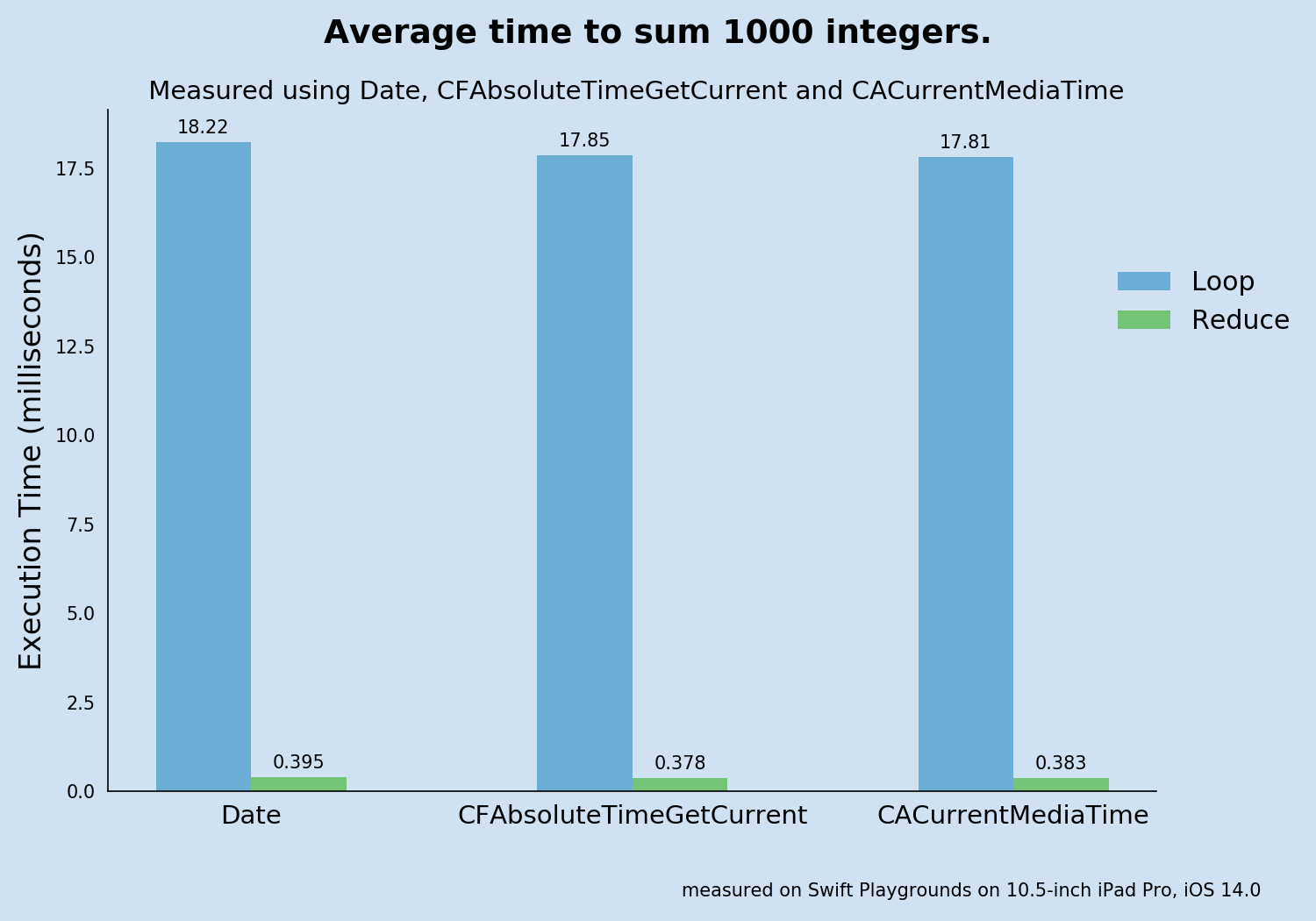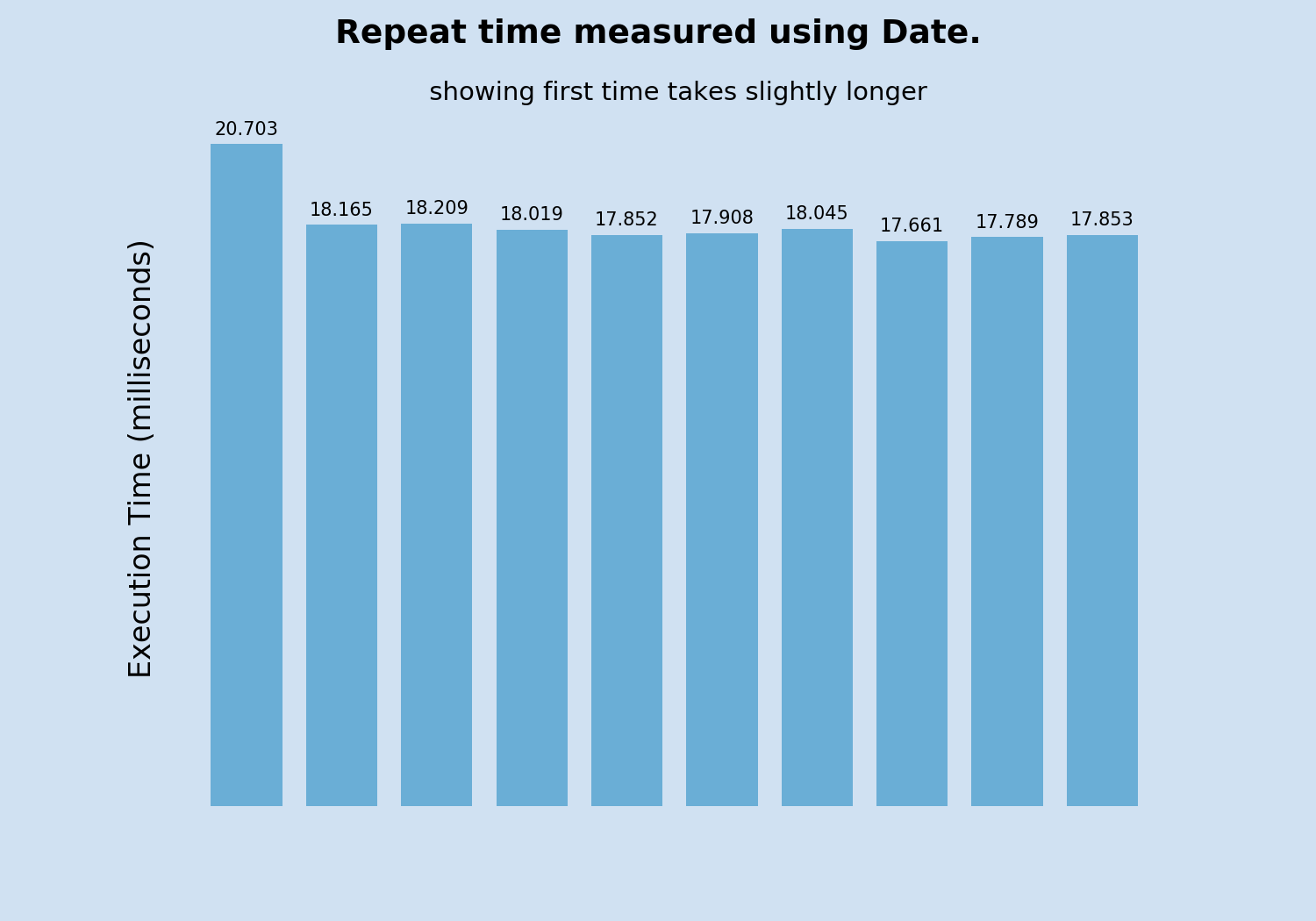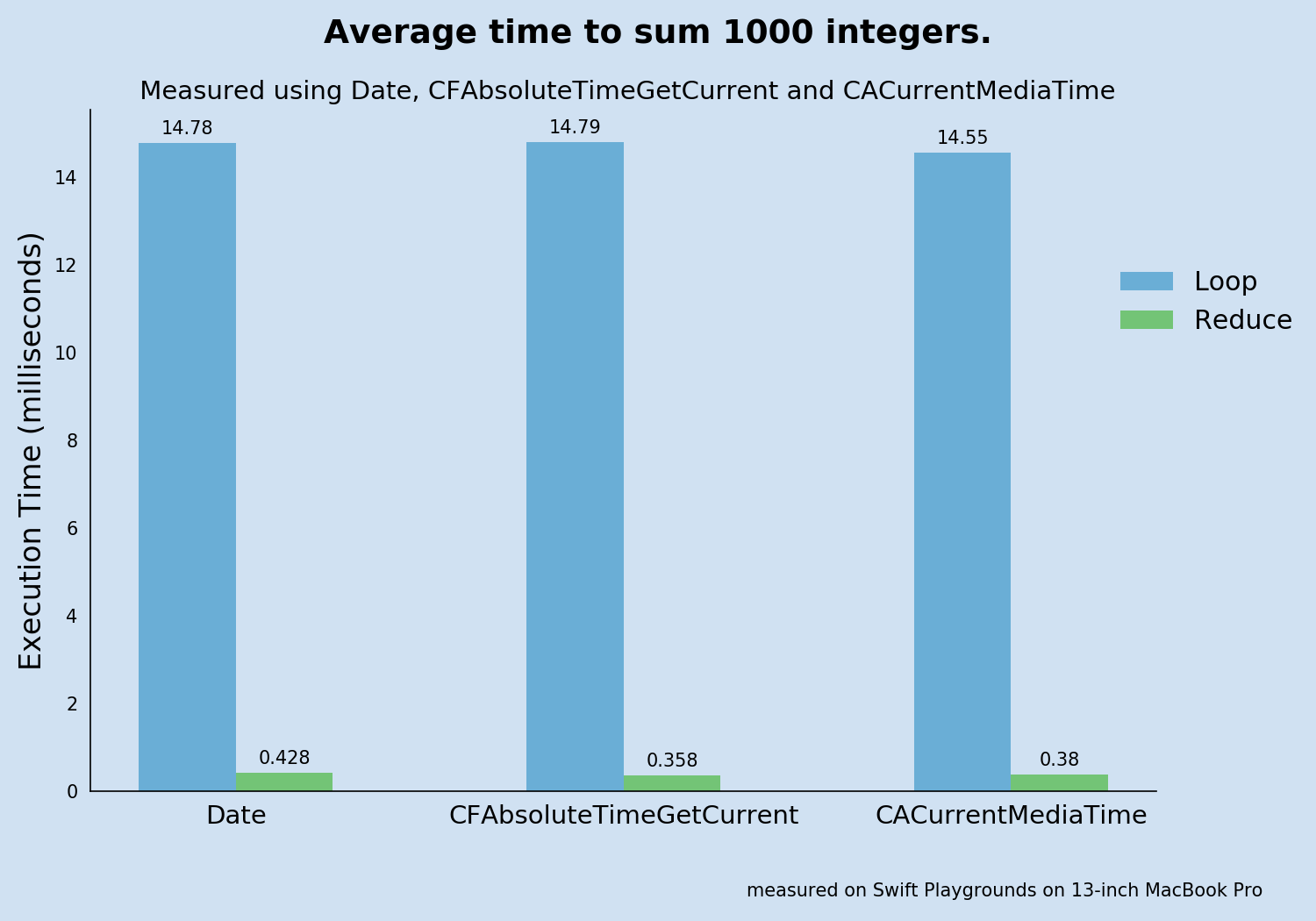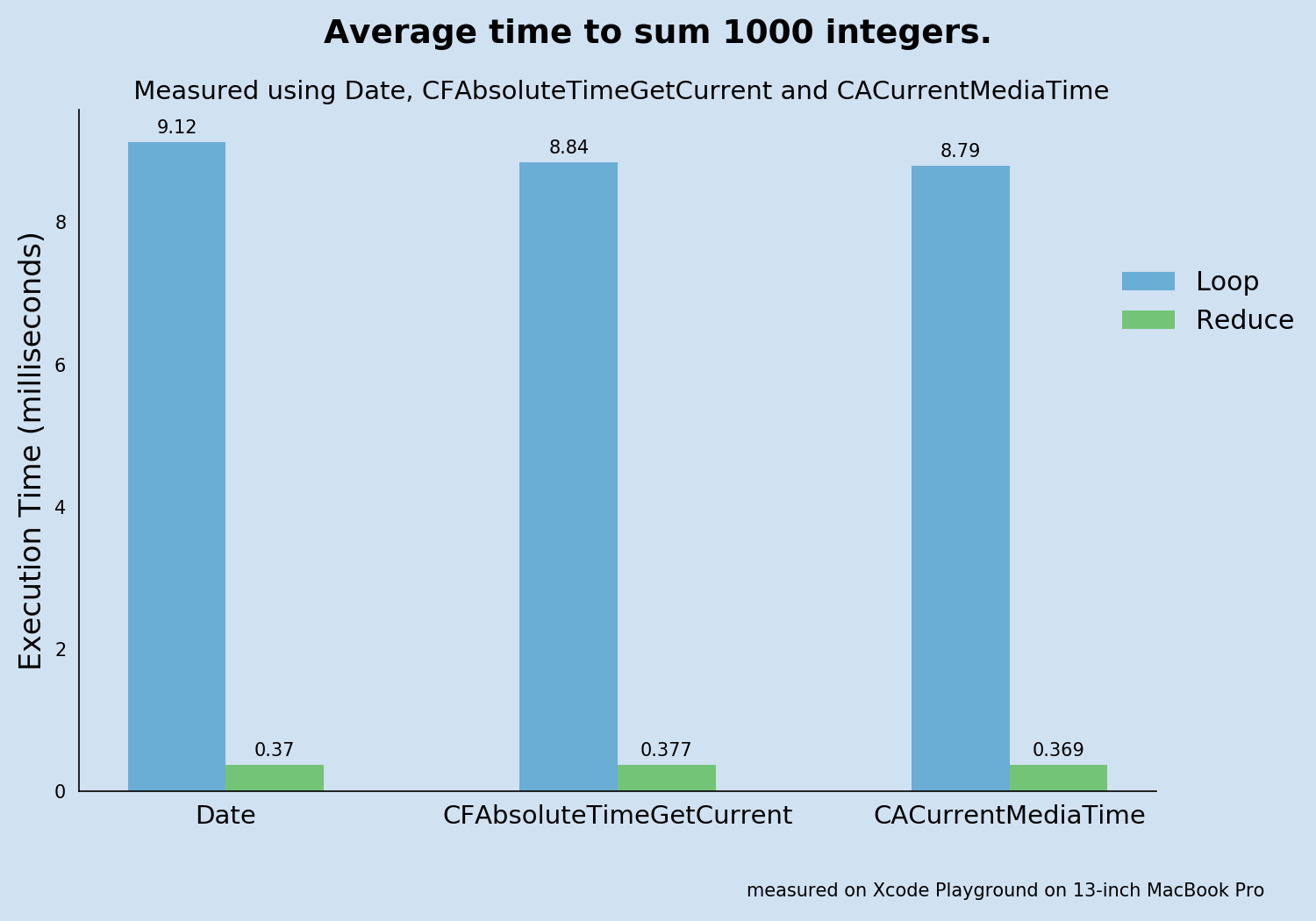# Timing Swift functions

It can be very useful to check the execution time of a group of swift statements or swift function when evaluating the most efficient way to perform a task. In this article three different mechanisms are used to measure the execution time to sum up 1000 random numbers.

The exact execution time of any function depends on many factors including hardware, operating system, and other operations currently in progress. Timing functions are used to get the time before anf after a function is run and to establish the duration of the function. The value is not in determining an exact execution time, but in being able to compare different methods to identify the more efficient. The speed of adding numbers in a list using a for loop is compared to using the reduce function.

## Create random list

Use a function to create a random list of integers between 1 and 1000 inclusive.

 1func createRandomList(_ n: Int) -> [Int] {
2    /// Returns a list of the specified size
3    /// containing random integers between 1 and 1000 inclusive
4    ///
5    /// The list may contain duplicate values
6    return (0..<n).map{_ in Int.random(in: 1...1000)}
7}
8
9// Test the function
10print("5 random numbers = \(createRandomList(5))")
11// 5 random numbers = [636, 321, 411, 966, 104]
12print("7 random numbers = \(createRandomList(7))")
13// 7 random numbers = [956, 848, 342, 903, 915, 938, 756]


Create a random list of 1000 numbers that can be used to time the summing functions. This ensures that the exact same list of numbers are used when comparing the functions.

1let randomList = createRandomList(1000)


## Create functions to sum up the list of integers

This function uses a for loop to add up all the numbers in the list.

 1func sumUsingLoop(_ n:[Int]) -> Int {
2    /// Add the list of integers using a for loop
3    /// Returns the sum of the list of integers
4    var result = 0
5    for i in n {
6        result += i
7    }
8    return result
9}
10
11// Test the function
12print("sum of numbers = \(sumUsingLoop([1,2,3,4,5]))")
13// sum of numbers = 15


This function uses the reduce method to add up all the numbers in the list.

1func sumUsingReduce(_ n:[Int]) -> Int {
2    /// Add the list of integers using reduce
3    /// Returns the sum of the list of integers
4    return n.reduce(0, +)
5}
6
7// Test the function
8print("sum of numbers = \(sumUsingReduce([1,2,3,4,5]))")
9// sum of numbers = 15


## Measuring Swift execution time

The three mechanisms used to measure Swift code execution time are:

### Date from Foundation

The Date structure represents a single point in time. It provides methods for comparing dates and calculating the time interval between two dates. This is used to set the date before and after calling the function and then getting the interval between these values.

The following function takes the number of repeats, the function to test and the list of integers. It calculates the average execution time by executing the function repeatedly, measuring each execution time and then averaging them. It returns a tuple containing the average execution time in milliseconds as well as the list of each execution time and the list of the function results. The function results were added to ensure the function was executing correctly.

 1import Foundation
2
3func timeFunctionUsingDate(repeats: Int, function: ([Int])->Int, list: [Int]) -> (Double, [Double], [Int]) {
4    /// Calculate the average execution time by executing the passed in function
5    /// with the specified list for the number of repeats specified.
6    /// Calculate the average execution time.
7    /// Uses Date to measure time duration.
8    ///
9    /// Returns a tuple containing the following:
10    ///   - average execution time in milliseconds
11    ///   - the list of each execution time in milliseconds
12    ///   - the list of the function results (to ensure these are the same)
13
14    var funcResults = [Int]()
15    funcResults.reserveCapacity(repeats)
16    var results = [Double]()
17    results.reserveCapacity(repeats)
18    var averageTime = 0.0
19    for _ in 1 ... repeats {
20        let startTime = Date()
21        funcResults.append(function(list))
22        let endTime = Date()
23        let timetaken = DateInterval(start: startTime, end: endTime)
24        results.append(timetaken.duration * 1000.0)
25    }
26    averageTime = results.reduce(0,+) / Double(results.count)
27    return (averageTime, results, funcResults)
28}


### CFAbsoluteTimeGetCurrent from time utilities

CFAbsoluteTimeGetCurrent returns the current system absolute time relative to the absolute reference date of Jan 1 2001. This may not be the most reliable method as repeated calls to this function do not guarantee monotonically increasing results.

The following function takes the number of repeats, the function to test and the list of integers. This is similar to the previous function except it uses CFAbsoluteTimeGetCurrent to measure the time taken.

 1func timeFunctionUsingAbsoluteTime(repeats:Int, function: ([Int])->Int, list:[Int]) -> (Double, [Double], [Int]) {
2    /// Calculate the average execution time by executing the passed in function
3    /// with the specified list for the number of repeats specified.
4    /// Calculate the average execution time.
5    /// Uses CFAbsoluteTimeGetCurrent to measure time duration.
6    ///
7    /// Returns a tuple containing the following:
8    ///   - average execution time in milliseconds
9    ///   - the list of each execution time in milliseconds
10    ///   - the list of the function results (to ensure these are the same)
11
12    var funcResults = [Int]()
13    var results:[Double] = []
14    var averageTime = 0.0
15    for _ in 1 ... repeats {
16        let startTime = CFAbsoluteTimeGetCurrent()
17        funcResults.append(function(list))
18        let endTime = CFAbsoluteTimeGetCurrent()
19        let timetaken = endTime - startTime
20        results.append(timetaken * 1000.0)
21    }
22    averageTime = results.reduce(0,+) / Double(results.count)
23    return (averageTime, results, funcResults)
24}


### CACurrentMediaTime from Core Animation

CACurrentMediaTime returns the current absolute time, in seconds. It is a wrapper around mach_absolute_time, considered the most accurate time function in the system. CACurrentMediaTime converts the data from mach_absolute_time to a human readable form.

The following function takes the number of repeats, the function to test and the list of integers. This is similar to the previous functions except it uses CACurrentMediaTime to measure the time taken.

 1
2import QuartzCore
3func timeFunctionUsingMediaTime(repeats:Int, function: ([Int])->Int, list:[Int]) -> (Double, [Double], [Int]){
4    /// Calculate the average execution time by executing the passed in function
5    /// with the specified list for the number of repeats specified.
6    /// Calculate the average execution time.
7    /// Uses CACurrentMediaTime to measure time duration.
8    ///
9    /// Returns a tuple containing the following:
10    ///   - average execution time in milliseconds
11    ///   - the list of each execution time in milliseconds
12    ///   - the list of the function results (to ensure these are the same)
13
14    var funcResults = [Int]()
15    funcResults.reserveCapacity(repeats)
16    var results = [Double]()
17    results.reserveCapacity(repeats)
18    var averageTime = 0.0
19    for _ in 1 ... repeats {
20        let startTime = CACurrentMediaTime()
21        funcResults.append(function(list))
22        let endTime = CACurrentMediaTime()
23        let timetaken = endTime - startTime
24        results.append(timetaken * 1000.0)
25    }
26    averageTime = results.reduce(0,+) / Double(results.count)
27    return (averageTime, results, funcResults)
28}


## Compare swift execution time

Use the functions above to measure the execution times. Initially run with 10 repeats.

 1repeatNum = 10
2
3let loop1 = timeFunctionUsingDate(repeats: repeatNum,
4                                  function: sumUsingLoop(_:),
5                                  list: randomList)
6
7let reduce1 = timeFunctionUsingDate(repeats: repeatNum,
8                                    function: sumUsingReduce(_:),
9                                    list: randomList)
10
11let loop2 = timeFunctionUsingAbsoluteTime(repeats: repeatNum,
12                                          function: sumUsingLoop(_:),
13                                          list: randomList)
14
15let reduce2 = timeFunctionUsingAbsoluteTime(repeats: repeatNum,
16                                            function: sumUsingReduce(_:),
17                                            list: randomList)
18
19let loop3 = timeFunctionUsingMediaTime(repeats: repeatNum,
20                                       function: sumUsingLoop(_:),
21                                       list: randomList)
22
23let reduce3 = timeFunctionUsingMediaTime(repeats: repeatNum,
24                                         function: sumUsingReduce(_:),
25                                         list: randomList)Average time to sum one thousand numbers Comparing speed of For loop versus Reduce function to sum one thousand numbers

It is clear that summing up the numbers using reduce is significantly faster than using a for loop. It appears that using reduce is almost 50 times faster regardless of which methon is used to measure the execution time.

 1print(String(format: "timeFunction Using Date:         loop = %.2f, reduce = %.3f, factor = %.0f",
2             loop1.0, reduce1.0, loop1.0/reduce1.0))
3print(String(format: "timeFunction Using AbsoluteTime: loop = %.2f, reduce = %.3f, factor = %.0f",
4             loop2.0, reduce2.0, loop2.0/reduce2.0))
5print(String(format: "timeFunction Using MediaTime:    loop = %.2f, reduce = %.3f, factor = %.0f",
6             loop3.0, reduce3.0, loop3.0/reduce3.0))
7
8// timeFunction Using Date:         loop = 18.22, reduce = 0.395, factor = 46
9// timeFunction Using AbsoluteTime: loop = 17.85, reduce = 0.378, factor = 47
10// timeFunction Using MediaTime:    loop = 17.81, reduce = 0.383, factor = 47

Measurement Mechanism Loop Time (milliseconds) Reduce Time (milliseconds) factor
Date 18.22 0.395 46
CFAbsoluteTimeGetCurrent 17.85 0.378 47
CACurrentMediaTime 17.81 0.383 47

### Closer look at using Date

The measured execution time seems slightly more when use the Date function from Foundation. These results are the average of 10 repeats. On closer examination of the individual measurements, it can be seen that the first time seems significantly longer than the other 9 measurements for Date. This could be caused by calling Date() for the first time, perhaps some initialisation is required. The average of the remaining timings, excluding the first one, is 17.94. This makes using Date much closer to results from CFAbsoluteTimeGetCurrent and CACurrentMediaTime.

Number Loop Time (seconds) Reduce Time (seconds)
1 20.703 0.372
2 18.165 0.405
3 18.209 0.373
4 18.019 0.381
5 17.852 0.396
6 17.908 0.405
7 18.045 0.397
8 17.661 0.403
9 17.789 0.407
10 17.853 0.412Repeat time to sum one thousand numbers using Date Repeat time measured using Date showing first time takes slightly longer

## Measurement on other hardware

The above results are from a Swift Playground on a 10.5-inch iPad Pro. The same playground was run using the Playgrounds app on 13-inch MacBook Pro.

#### Performance of For loop versus Reduce function to sum one thousand numbers on MacBook Pro

Measurement Mechanism Loop Time (milliseconds) Reduce Time (milliseconds) factor
Date 14.78 0.428 35
CFAbsoluteTimeGetCurrent 14.79 0.358 41
CACurrentMediaTime 14.55 0.380 38Average time to sum one thousand numbers using Playgrounds on MacBook Pro Comparing speed on Playgrounds on MacBook Pro

The loop time is faster, but the reduce time has not changed much. It should be noted that these execution times are in milliseconds and the documentation does not recommend using functions like Date() for accuracy at less than a millisecond. All the measurements for the reduce function are less than half of a millisecond.

The same code was also run on a Playground in Xcode

#### Performance of For loop versus Reduce function to sum one thousand numbers on MacBook Pro

Measurement Mechanism Loop Time (milliseconds) Reduce Time (milliseconds) factor
Date 9.12 0.370 25
CFAbsoluteTimeGetCurrent 8.84 0.377 23
CACurrentMediaTime 8.79 0.369 24Average time to sum one thousand numbers using Xcode on MacBook Pro Comparing speed on Xcode Playground on MacBook Pro

## Conclusion

Any of the three mechanisms can be used to measure execution time of a function. These measurements are used to determine which function implementation is better rather than determining the exact execution time. The measurement of execution time can provide great insight on which algorithm to use and help improve performance of Swift apps.

The hardware and environment does influence the performance and the loop time did improve when the same playground was run on a MacBook Pro as opposed to an iPad Pro. The reduce time did not seem to change much, which could be due to the time being less than half of a millisecond.

Is is important when comparing the performance of different functions that the comparison is done in the same environment. Finally the reduce function is significantly faster than using a loop in any environment.